# KSEEB Solutions for Class 9 Maths Chapter 10 Linear Equations in Two Variables Ex 10.3

KSEEB Solutions for Class 9 Maths Chapter 10 Linear Equations in Two Variables Ex 10.3 are part of KSEEB Solutions for Class 9 Maths. Here we have given Karnataka Board Class 9 Maths Chapter 10 Linear Equations in Two Variables Exercise 10.3.

## Karnataka Board Class 9 Maths Chapter 10 Linear Equations in Two Variables Ex 10.3

Question 1.
Draw the graph of each of the following linear equations in two variables :
i) x + y = 4
ii) x – y = 2
iii) y = 3x
iv) 3 = 2x + y
Solution:
i) x + y = 4
This can be written in the form of y = mx + c, then
y = 4 – x.

 x 0 1 4 -1 y 4 3 0 5ii) x – y = 2
-y = 2 – x
y = -2 + x
y = x – 2

 x 0 1 2 4 y -2 -1 0 2iii) y = 3x

 x 0 1 2 -1 y 0 3 6 -3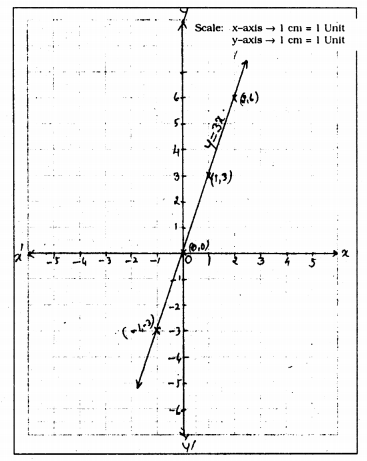iv) 3 = 2x + y
2x + y = 3
y = 3 – 2x

 x 0 1 2 -1 y 3 1 -1 5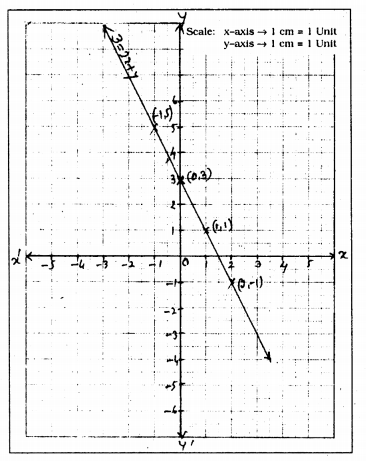Question 2.
Give the equations of two lines passing through (2, 14). How many more such lines are there, and why ?
Solution:
E.g. i) y = 7x
ii) x = $$\frac{y}{7}$$
iii) x + y = 16 .
We may write infinite equation, because Graph of a linear equation in two variables has many solutions.

Question 3.
If the point (3, 4) lies on the graph of the equation 3y = ax + 7, find the value of a.
Solution:
3y = ax + 7
If point (3, 4) then a = ?
3y = ax + 7
3(4) = a(3) + 7 ∵ x = 3, y = 4
12 = 3a + 7
3a + 7 = 12
3a = 12 – 7
3a = 5
∴ a = $$\frac{5}{3}$$

Question 4.
The taxi fare in a city is as follows:
For the first kilometre, the fare is Rs. 8 and for the subsequent distance, it is Rs. 5 per km. Taking the distance covered as x km and the total fare is Rs. y. write a linear equation for this information, and draw its graph.
Solution:
Let the distance travelled be x km.
Let the total distance be y km.For the first kilometre, the fare is Rs. 8 + fare for the remaining distance, i.e. (x – 1) km.
Rs. 5 for 1 km.
if it travels (x – 1) km … ? Rs.
Rs. (x – 1)5
∴ Total cost = Rs. y
Fare for first km + fare for subsequent distance means (x-1) km = Rs. y
8 + (x – 1)5 = y
8 + 5x – 5 = y
8 – 5 + 5x = y
3 + 5x = y
∴ y = 3 + 5x linear equation.

 x 0 1 -1 -2 y 3 8 -2 -7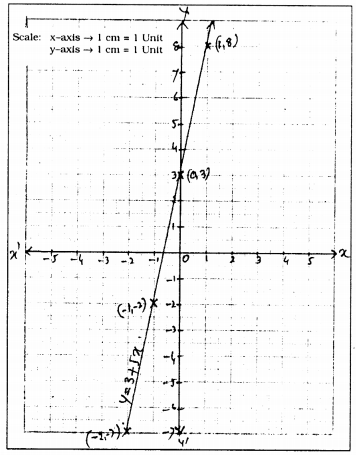Question 5.
From the choices given below, choose the equation whose graphs are given in Fig. 10.6 and Fig. 10. 7.Solution:
Solution for graph of linear equation of Fig. 10.6 :Sum of (x + y) = 0.
Equation for this is

(ii) x + y = 0
because x = -y we have OR -x = y
0 = 0
1 = -1
-1 = 1
Solutions for graph for linear equation in Fig. 10.7 :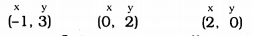x + y = 2 is common in all.
∴ y = -x + 2
∴ Ans: (iii) y = -x + 2 equation.

Question 6.
If the work done by a body on application of a constant force is directly proportional to the distance travelled by the body, express this in the form of an equation in two variables and draw the graph of the same by taking the constant force as 5 units. Also read from the graph the work done when the distance travelled by the body is
(i) 2 units
(ii) 0 unit.
Solution:
Let the work done be, W
Distance travelled be D.
If constant force is K, then
Force is directly proportional to the distance travelled by the body.
∴ W ∝ D.
W = K × D
Constant force, K = 5 units.
W = 5 × D linear equation.
If D = 2, then W = 5D
W = 5 × 2
W = 10
If D = 0, then W = 5D
W = 5 × 0
W = 0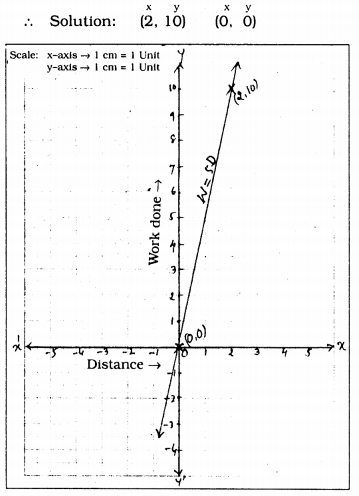Question 7.
Yamini and Fatima, two students of Class IX of a school, together contributed Rs. 100 towards the Prime Minister’s Relief Fund to help the earthquake victims. Write a linear equation which satisfies this data. (You may take their contributions as Rs. x and Rs. y.) Draw the graph of the same.
Solution:
Yamini and Fatima together contributed Rs. 100 towards the Prime Minister’s Relief Fund to help the earthquake victims.
In that contribution of Yamini is R. x Contribution of Fatima is Rs. y Together Rs. 100.
∴ x + y = 100
∴ y = 100 – x.

 x 20 40 50 60 y 80 60 50 40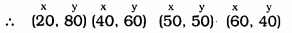Question 8.
In countries like USA and Canada, the temperature is measured in Fahrenheit, whereas in countries like India, it is measured in Celsius. Here is a linear equation that converts Fahrenheit to Celsius :
F = $$\left(\frac{9}{5}\right)$$ C + 32.
(i) Draw the graph of the linear equation above using Celsius for x-axis and Fahrenheit for the y-axis.
(ii) If the temperature is 30° C, what is the temperature in Fahrenheit?
(iii) If the temperature is 95° F, what is the temperature in Celsius?
(iv) If the temperature is 0° C, what is the temperature in Fahrenheit, and if the temperature is 0° F, what is the temperature in Celsius?
(v) Is there a temperature which is numerically the same in both Fahrenheit and Celsius? If yes, find it.
Solution:
Let Temperature be Celsius C,
The temperature be Fahrenheit F.
F = $$\left(\frac{9}{5}\right)$$ C + 32

 C 10 30 0 35 F 50 86 32 95

In the x-axis Celsius,
In the y-axis Fahrenheit.
(i) Graph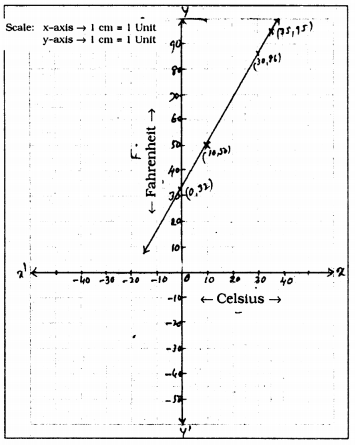(ii) If temperature is 30° C, then 86° F.
(iii) If the temperature is 95° F, then 35° C.
(iv) If temperature is 0° C, then 32° F.
If 0°F, then -32°C
(v) Temperature is not equal in Celsius and Fahrenheit.
because C ≠ F

We hope the KSEEB Solutions for Class 9 Maths Chapter 10 Linear Equations in Two Variables Ex 10.3 help you. If you have any query regarding Karnataka Board Class 9 Maths Chapter 10 Linear Equations in Two Variables Exercise 10.3, drop a comment below and we will get back to you at the earliest.

error: Content is protected !!